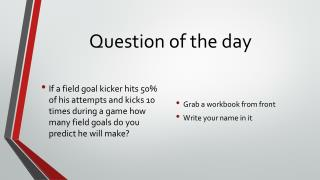DownloadDownload PresentationQuestion of the day

# Question of the day

Download Presentation## Question of the day

- - - - - - - - - - - - - - - - - - - - - - - - - - - E N D - - - - - - - - - - - - - - - - - - - - - - - - - - -
##### Presentation Transcript

1. Question of the day • If a field goal kicker hits 50% of his attempts and kicks 10 times during a game how many field goals do you predict he will make? • Grab a workbook from front • Write your name in it

2. Agenda Go over lesson 8 Notes on prediction Lesson in workbook Exit ticket

3. Review from yesterday • We can use one experimental probability to solve another experimental probability by writing a proportion • We can use theoretical probability to predict experimental probability by writing a proportion • = • If I roll a die 5 times and get a 3 twice how many times would you predict getting a 3 if you rolled it 15 times? • If you flip a coin 40 times how many heads would you predict to land on?

4. remember • Remember how to turn percent into fraction. • 40% = = • 32% = =

5. remember • How to change percent into decimal • Divide by 100 • 70% = .7 • 4% = .04 • 34% = .34

6. Using estimation for experimental probability • If we know one experimental probability then we can use it to predict a probability in the future • Try it with equations • Tim finds his experimental probability of getting a hit is 40%. Out of 350 at-bats, how many times can he expect to get a hit? • Write an equation • What is 40% of 350? • 40% = .4 and of means multiply • 350 x .4 = 140

7. Same idea with proportions • This time we can use the proportion idea we used yesterday • Tim finds his experimental probability of getting a hit is 40%. Out of 350 at-bats, how many times can he expect to get a hit? • 40% = • =

8. Assignment Workbook p. 503 Also workbook pages 499-501 have some good notes for this topic

9. Exit ticket Marty makes his field goals 74% of the time. Out of 150 attempts how many times can he be expected to make a field goal?